# Filling circles

Today's blog post comes from the stream of image processing questions over at MATLAB Answers.

I thought it might be worth a little discussion here (plus, I wanted to point out the value of MATLAB Answers.)

Typically, the process of answering the question started out with trying to understand the question better. "What does remove mean to you?" asked Image Analyst. The answer came back, "I want to make the pixels in the circle be black."

OK, let's give that a try.

I = imread('coins.png');
imshow(I)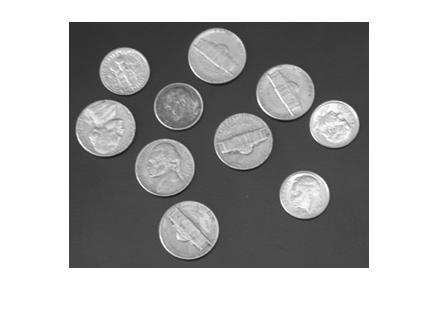(Hmm. Guess I didn't have any quarters in my pocket when I took this picture.)

Suppose we want to set to black all the pixels centered at (X = 175, Y = 120) and with radius 35.

I like Image Analyst's recommended procedure:

1. Compute a mask image. Generally, the term mask or mask image means a binary image whose foreground pixels correspond to a set of pixels of interest.
2. Use logical indexing with the mask image to modify the desired pixels.

xc = 175;
yc = 120;
r = 33;

x = 1:size(I,2);
y = 1:size(I,1);
[xx,yy] = meshgrid(x,y);

mask = hypot(xx - xc, yy - yc) <= r;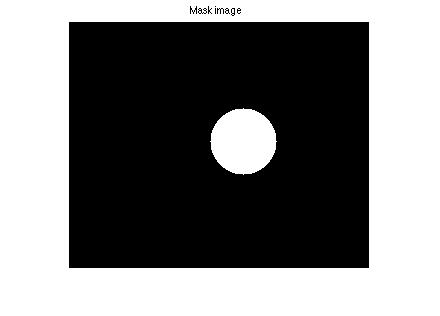Next, use logical indexing to modify the pixels of I corresponding to the foreground pixels of mask.

I2 = I;
imshow(I2)
title('Image with some pixels set to black')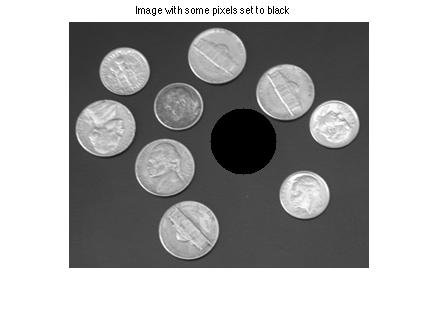And we just lost 5 cents.

Image Analyst pointed out that you can use this technique to set pixel values in the circle to any value you want. How about white:

I2(mask) = 255;
imshow(I2)
title('Image with some pixels set to white')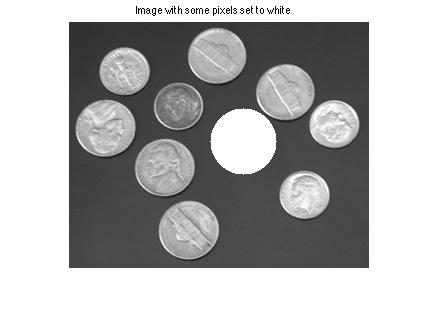For fun, how about setting the pixels to the background color. How do we determine that? I might start by thresholding the original image.

bw = im2bw(I,graythresh(I));
imshow(bw)
title('Thresholded image')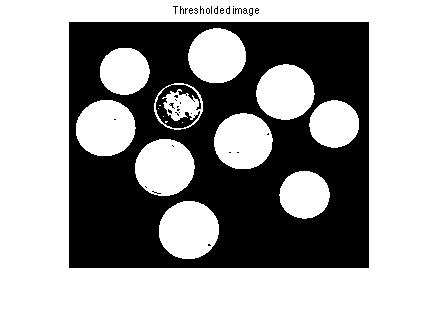Now fill in the holes.

bw = imfill(bw,'holes');
imshow(bw)
title('Thresholded image with holes filled')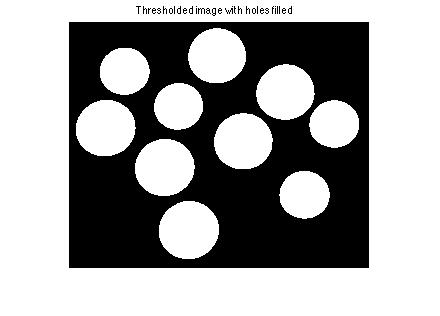Compute the mean value of the background pixels (using logical indexing again!).

bg_mean = mean(I(~bw))

bg_mean =

66.9959



Replace the pixels in the circle with the computed average background value.

I2(mask) = bg_mean;
imshow(I2)
title('Circle pixels filled with average background')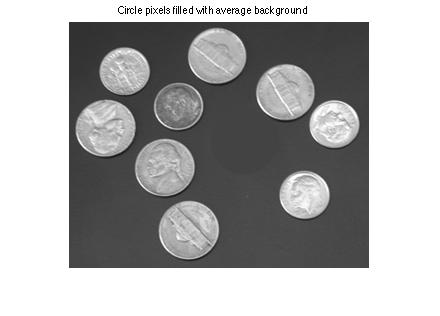Let me point out two related Image Processing Toolbox functions. The roifill function can fill a region based on a mask; it fills pixels "smoothly" from the adjacent background pixels.

I3 = roifill(I,mask);
imshow(I3)
title('Circle pixels replaced using roifill')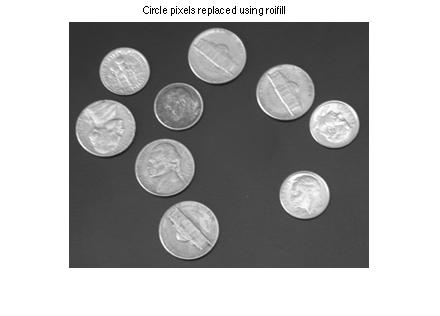I also want to mention imellipse. You can use this function to draw circles interactively using the mouse. Then you can use the createMask function to get the mask image corresponding to the circle you drew.

Happy circle filling!

Published with MATLAB® R2014a

|